## Year 4: Revise adding to 50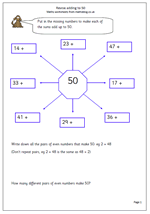Here is a snappy little worksheet to check that children can add on from 2-digit numbers to make 50. There are several different mental strategies that can be used, but the most efficient is usually to count on to the next whole ten and then count on in tens to 50.

Watch out for a common error whereby children add on to make 10 and then add on again from the original tens number; this will give them a total 10 more than is correct.

## Year 6 revision: More rounding millions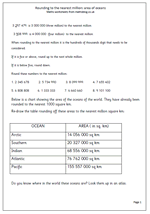Here we have another in our series of rounding larger numbers to the nearest million. This page looks in particular at the world’s oceans and the numbers involved have already been rounded to the nearest 1 000 sq km.

Children need to be confident when dealing with larger numbers, but this will only happen if they are secure in their knowledge of place value and how to read and write numbers. It may well be worth going back over this before attempting rounding.

Round to million  (oceans)

## Coming soon: Rounding, addition and doublingComing soon we have another in our series of rounding larger numbers to the nearest million. This page will look in particular at the world’s oceans and the numbers involved have already been rounded to the nearest 1 000 sq km.

We will also be publishing a snappy little worksheet to check that children can add on from 2-digit numbers to make 50. There are several different mental strategies that can be used, but the most efficient is usually to count on to the next whole ten and then count on in tens to 50.

Doubling 2-digit numbers can be quite easy or fairly tricky; it all depends on the numbers, so look out for a page on doubling next week.

## Year 3 maths worksheets: Subtraction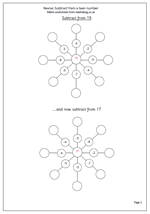Many children still need plenty of practice with adding and subtracting small numbers mentally. Here is a nifty way to give practice in subtracting a single digit from a teen in a slightly different way from the usual questions.Suitable for bright year 2 or year 3 children.

The second page shows a blank grid which can be printed out and your own choice of numbers put in – useful for children with particular problems with subtraction.

These pages can be found in our Year 3, Know Number facts section

Subtract from a teen

## Resource of the Week: Number squares for year 2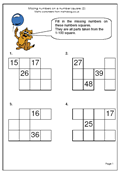This wek we are highlighting a Year 2 worksheet on number squares. This is our second in a series for year 2 children where they have to complete the missing numbers in a 100 square. Some of the squares have been removed from the rectangles which, curiously, seems to make this task harder than when working with a complete rectangle. This is good practice for children who are not confident with counting up to 100. Don’t be surprised if children stumble over counting up to 100 out loud. It is something we often take for granted, but of course, has to be learned.

Thanks to URBrainy for letting me use this page. They have thousands of worksheets for Key Stage 1 and you can try it out for nothing here.

Missing numbers on a number (p 2)

## Year 6 revision: Rounding millions (1)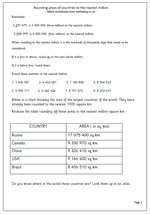Many children in Year 6 have difficulty reading and writing large numbers, including millions. This page is all based on millions as it looks at the area in square kilometres of some of the largest countries in the world. The task is to round the numbers to the nearest million, and as it happens all of these need to be rounded down.

It is well worth ‘talking through’ this page to make sure that the numbers are read correctly.

Round to million 1 (countries)

## Year 4 maths worksheet: multiples of 10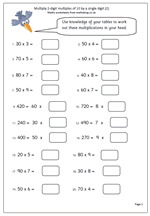The summer holidays are drawing to a close so what better for all those children just entering year 4 than a nice little mental arithmetic page. This page looks again at multiplying 2-digit multiples of 10 by a single digit. Dead easy if tables are known. If tables are not known this becomes much more difficult, so it is a good page to give to check that tables are sharp!

This page and many similar can be found in our Year 4 maths resources, Knowing Number Facts.

Multiply 2-digit multiples of 10_(p2)

## Coming soon: multiplication, rounding and subtractionWith the new term looming why not get in some early maths practice?  Next week we look again at multiplying 2-digit multiples of 10 by a single digit. Dead easy if tables are known.

Many children in Year 6 have difficulty reading and writing large numbers, including millions. We will be publishing a great page which looks at the area in square kilometres of some of the largest countries in the world.

Younger children still need plenty of practice with adding and subtracting small numbers mentally. We will show a nifty way to give practice in subtracting a single digit from a teen in a slightly different way from the usual questions. Suitable for bright year 2 or year 3 children.

## Year 5 Calculator game: 8 times table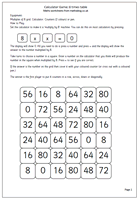This page is another in our calculator series, this time for the 8 times table. The grid has a selection of multiples of 8 up to 10 x 8. The idea is to play with a partner, choose a square and then enter a number on the calculator that you think will produce the number in the square when multiples by 8. if successful place a counter on the square. The aim is to try and get four in a row.

Remember that if the 8 times table is not known, multiplying by 8 can be achieved by doubling, doubling and doubling again.

This page can be found in our year 5 section under Knowing Number Facts

8x table calculator game

## Resource of the Week: Year 4 division problems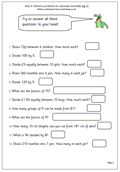This page has a range of different ways of asking division questions which children need to be able to recognise and there are a number  of methods that can be used to work out the answers mentally.

It is important the tables are known so that the facts can be used. For example if it is known that 6 x 6 = 36, then 6 x 60 can be quickly worked out as 360, and in turn 360 divided by 6 is 60.

Another useful tip is when dividing by 5 mentally it is often possible to divide by 10 and then double the answer.